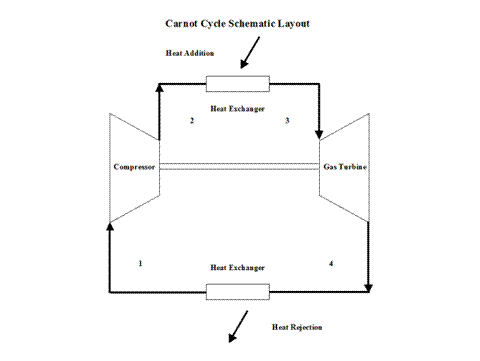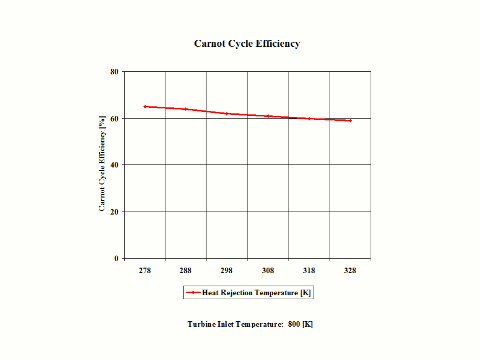top of page

### Carnot Cycle Analysis -- Free One (1) Credit Hour of CE

Carnot Cycle

Introduction

This section provides a Carnot Cycle analysis when the working fluid is air.

Analysis

In the presented Carnot Cycle analysis, only air is considered as the working fluid behaving as a perfect gas -- specific heat has a constant value.  Ideal gas state equation is valid -- pv = RT.

Air enters a compressor at point 1 and it exits the compressor at point 2.  Isentropic compression is considered with no entropy change.  Air enters a heat exchanger -- heat addition -- at point 2 and it exits the heat exchanger at point 3.  At a constant temperature, heat addition takes place.  Air enters a turbine at point 3 and it exits the turbine at point 4.  Isentropic expansion is considered with no entropy change.  Air enters a heat exchanger -- heat rejection -- at point 4 and it exits the heat exchanger at point 1.   At a constant temperature, heat rejection takes place.  It should be mentioned that air at point 1 enters the compressor and the cycle is repeated.

Figure 1 contains a Carnot Cycle schematic layout.Figure 1 - Carnot Cycle Schematic Layout

Figure 2 presents a Carnot Cycle entropy vs temperature diagram.Figure 2 - Carnot Cycle Temperature vs Entropy Diagram

Figure 3 presents the Carnot Cycle efficiency as a function of the heat addition temperature.  It should be noted that the inlet conditions are standard ambient conditions:  temperature of 298 [K] and absolute pressure of 1 [atm].Figure 3 - Carnot Cycle Efficiency vs Heat Addition Temperature

Figure 4 presents the Carnot Cycle efficiency as a function of the heat rejection temperature.  It should be noted that the turbine inlet temperature is at 800 [K].Figure 4 - Carnot Cycle Efficiency vs Heat Rejection Temperature

One can notice that the Carnot Cycle efficiency increases with an increase in the heat addition temperature when the heat rejection temperature does not change at all.  One can notice that the Carnot Cycle efficiency decreases with an increase in the heat rejection temperature when the heat addition temperature does not change at all.

Assumptions

Working fluid is air.  There is no friction.  Compression and expansion are isentropic -- there is no entropy change.  During heat addition and heat rejection, the air temperature does not change.  Ideal gas state equation is valid -- pv = RT.  Air behaves as a perfect gas -- specific heat has a constant value.

Governing Equations

T2/T1 = (p2/p1)^(ϰ-1)/ϰ

T3/T4 = (p3/p4)^(ϰ-1)/ϰ
ϰ = cp/cv
c
p - cv = R
pv = RT
efficiency = 1 - T
1/T2
efficiency = 1 - T
R/TA

Input Data

T1 = 298 [K]
p
1 = 1 [atm]
R = 0.2867 [kJ/kg*K]
c
p = 1.004 [kJ/kg*K]
ϰ = 1.4 [/]

Results

Carnot Cycle Efficiency vs Heat Addition Temperature
Heat Rejection Temperature = 298 [K]

Temperature
[K]

500

600

700

800

900

1,000

Carnot Cycle
Efficiency
[%]

40

50

57

62

66

70

Carnot Cycle Efficiency vs Heat Rejection Temperature
Heat Addition Temperature = 800 [K]

Heat Rejection
Temperature
[K]

278

288

298

308

318

328

Carnot Cycle
Efficiency
[%]

65

64

62

61

60

59

Conclusions

The Carnot Cycle efficiency increases with an increase in the heat addition temperature when the heat rejection temperature does not change at all.   Furthermore, the Carnot Cycle efficiency decreases with an increase in the heat rejection temperature when the heat addition temperature does not change at all.  The Carnot Cycle efficiency is not dependent on the working fluid properties.

References

JANAF Thermochemical Data - Tables, 1970

bottom of page